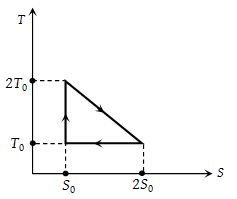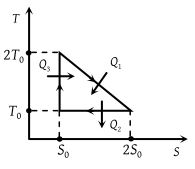# NEET Physics Thermodynamics Questions Solved

The temperature-entropy diagram of a reversible engine cycle is given in the figure. Its efficiency is(1) 1/3

(2) 2/3

(3) 1/2

(4) 1/4

(1) ${Q}_{1}={T}_{0}{S}_{0}+\frac{1}{2}{T}_{0}{S}_{0}=\frac{3}{2}{T}_{0}{S}_{0}$${Q}_{2}={T}_{0}{S}_{0}$ and ${Q}_{3}=0$

$\eta =\frac{W}{{Q}_{1}}=\frac{{Q}_{1}-{Q}_{2}}{{Q}_{1}}$

$=1-\frac{{Q}_{2}}{{Q}_{1}}=1-\frac{2}{3}=\frac{1}{3}$

Difficulty Level:

• 44%
• 23%
• 30%
• 4%
Crack NEET with Online Course - Free Trial (Offer Valid Till August 25, 2019)